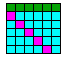##Lecture 7. Model of Leslie

Course: Quantitative Population Ecology
Dept. of Entomology, Virginia Tech, Blacksburg, VA

The model of Leslie is one of the most healivy used models in population ecology. This is a discrete-time model of an age-structured population which describes development, mortality, and reproduction of organisms. The model is formulated using linear algebra. This model is mostly used to answer the following two questions:

1. What is the rate of exponential growth (intrinsic rate of increase)?
2. What is the proportion of each age class in the stable age distribution?

Alexei Sharov 1/12/96